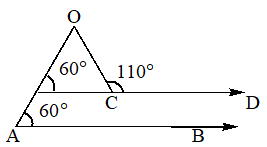In the given figure, AB||CD. If ∠BAO=60° and ∠OCD=110°, then ∠AOC=

# In the given figure, $AB||CD.$ If $\angle BAO=60°$ and $\angle OCD=110°,$ then $\angle AOC=$1. A

$70°$

2. B

$60°$

3. C

$50°$

4. D

$40°$

Register to Get Free Mock Test and Study Material

+91

Live ClassesRecorded ClassesTest SeriesSelf Learning

Verify OTP Code (required)

### Solution:

$x=110°-60°=50°$

## Related content

 Matrices and Determinants_mathematics Critical Points Solved Examples Type of relations_mathematics